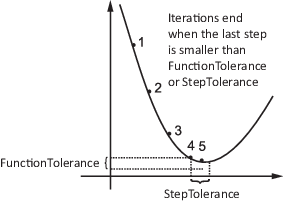## Tolerances and Stopping Criteria

The number of iterations in an optimization depends on a solver's stopping criteria. These criteria include several tolerances you can set. Generally, a tolerance is a threshold which, if crossed, stops the iterations of a solver.

Set tolerances and other criteria using `optimoptions` as explained in Set and Change Optimization Options.

Tip

Generally set tolerances such as `OptimalityTolerance` and `StepTolerance` to be well above `eps`, and usually above `1e-14`. Setting small tolerances does not always result in accurate results. Instead, a solver can fail to recognize when it has converged, and can continue futile iterations. A tolerance value smaller than `eps` effectively disables that stopping condition. This tip does not apply to `fzero`, which uses a default value of `eps` for the `TolX` tolerance.

`optimoptions` displays tolerances. For example,

```options = optimoptions('fmincon'); [options.OptimalityTolerance,options.FunctionTolerance,options.StepTolerance]```
```ans = 1.0e-06 * 1.0000 1.0000 0.0001```

You can also find the default tolerances in the options section of the solver function reference page.

• `StepTolerance` is a lower bound on the size of a step, meaning the norm of (xi – xi+1). If the solver attempts to take a step that is smaller than `StepTolerance`, the iterations end. `StepTolerance` is generally used as a relative bound, meaning iterations end when |(xi – xi+1)| < `StepTolerance`*(1 + |xi|), or a similar relative measure. See Tolerance Details.• For some algorithms, `FunctionTolerance` is a lower bound on the change in the value of the objective function during a step. For those algorithms, if |f(xi) – f(xi+1)| < `FunctionTolerance`, the iterations end. `FunctionTolerance` is generally used as a relative bound, meaning iterations end when |f(xi) – f(xi+1)| < `FunctionTolerance`*(1 + |f(xi)|), or a similar relative measure. See Tolerance Details.

Note

Unlike other solvers, `fminsearch` stops when it satisfies both `TolFun` (the function tolerance) and `TolX` (the step tolerance).

• `OptimalityTolerance` is a tolerance for the first-order optimality measure. If the optimality measure is less than `OptimalityTolerance`, the iterations end. `OptimalityTolerance` can also be a relative bound on the first-order optimality measure. See Tolerance Details. First-order optimality measure is defined in First-Order Optimality Measure.

• `ConstraintTolerance` is an upper bound on the magnitude of any constraint functions. If a solver returns a point x with c(x) > `ConstraintTolerance` or |ceq(x)| > `ConstraintTolerance`, the solver reports that the constraints are violated at x. `ConstraintTolerance` can also be a relative bound. See Tolerance Details.

Note

`ConstraintTolerance` operates differently from other tolerances. If `ConstraintTolerance` is not satisfied (i.e., if the magnitude of the constraint function exceeds `ConstraintTolerance`), the solver attempts to continue, unless it is halted for another reason. A solver does not halt simply because `ConstraintTolerance` is satisfied.

• `MaxIterations` is a bound on the number of solver iterations. `MaxFunctionEvaluations` is a bound on the number of function evaluations. Iterations and function evaluations are discussed in Iterations and Function Counts.

There are two other tolerances that apply to particular solvers: `TolPCG` and `MaxPCGIter`. These relate to preconditioned conjugate gradient steps. For more information, see Preconditioned Conjugate Gradient Method.

There are several tolerances that apply only to the `fmincon` interior-point algorithm. For more information, see Interior-Point Algorithm in `fmincon` `options`.

There are several tolerances that apply only to `intlinprog`. See Some “Integer” Solutions Are Not Integers and Branch and Bound.